Home | | Statistics 12th Std | Chi-Square Distribution and Its Applications

# Chi-Square Distribution and Its Applications

General Procedure for Chi-Square Distribution and Its Applications: Properties, Procedure Steps, Example Solved Problems

CHI-SQUARE DISTRIBUTION AND ITS APPLICATIONS

Karl Pearson (1857-1936) was a English Mathematician and Biostatistician. He founded the worldŌĆÖs first university statistics department at University College, London in 1911. He was the first to examine whether the observed data support a given specification, in a paper published in 1900. He called it ŌĆśChi-square goodness of fitŌĆÖ test which motivated research in statistical inference and led to the development of statistics as separate discipline.

## 1. Chi-Square distribution

The square of standard normal variable is known as a chi-square variable with 1 degree of freedom (d.f.). Thus

If X ~ N (┬Ą, Žā2), then it is known that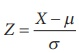~  N 0,1 . Further Z2 is said to follow Žć2 ŌĆō distribution with 1 degree of freedom (Žć2 ŌĆō pronounced as chi-square)

Note: i) If Xi ~ N (┬Ą, Žā2) , i = 1, 2, ŌĆ”, n are n iid random variables, then## 2. Properties of c2 distribution

It is a continuous distribution.

┬Ę              The distribution has only one parameter i.e. n d.f.

┬Ę              The shape of the distribution depends upon the d.f, n.

┬Ę              The mean of the chi-square distribution is n and variance 2n

┬Ę              If U and V are independent random variables having Žć2 distributions with degree of freedom n1 and n2 respectively, then their sum U + V has the same Žć2 distribution with d.f n1 + n2.

## 3. Applications of chi-square distribution

To test the variance of the normal population, using the statistic in note (ii)

┬Ę              To test the independence of attributes.

┬Ę              To test the goodness of fit of a distribution.

┬Ę              The sampling distributions of the test statistics used in the last two applications are approximately chi-square distributions.

## 4. Test of Hypotheses for population variance of the normal population (Population mean is assumed to be unknown)

Procedure

Step 1 : Let ┬Ą and Žā2 be respectively the mean and the variance of the normal population under study, where Žā2 is known and ┬Ą unknown. If Žā02 is an admissible value of Žā2, then frame the

Null hypothesis as H0: Žā 2 = Žā 02 and choose the suitable alternative hypothesis from

(i) H1 : Žā2 ŌēĀ Žā02 (ii) H1 : Žā2 > Žā02 (iii) H1 : Žā2 < Žā02

Step 2 : Describe the sample/data and its descriptive measures. Let (X1, X2, ŌĆ”, Xn) be a random sample of n observations drawn from the population, where n is small (n < 30).

Step 3 : Fix the desired level of significance ╬▒.

Step 4 : Consider the test statisticunder H0. The approximate sampling distribution of  the test statistic under H0 is the chi-square distribution with (nŌĆō1) degrees of freedom.

Step 5 : Calculate the value of the of Žć2 for the given sample asStep 6 : Choose the critical value of Žće2 corresponding to ╬▒ and H1 from the following table.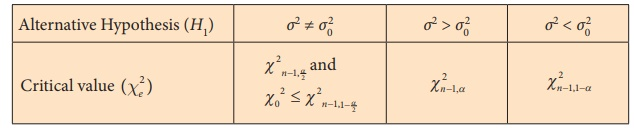Step 7 : Decide on H0 choosing the suitable rejection rule from the following table corresponding to H1Example 2.6

The weights (in kg.) of 8 students of class VII are 38, 42, 43, 50, 48, 45, 52 and 50. Test the hypothesis that the variance of the population is 48 kg, assuming the population is normal and ┬Ą is unknown.

Solution:

Step 1 : Null Hypothesis H0: Žā2 = 48 kg.

i.e. Population variance can be regarded as 48 kg.

Alternative hypothesis H1: Žā2 ŌēĀ 48 kg.

i.e. Population variance cannot be regarded as 48 kg.

Step 2 : The given sample information is

Sample size (n)= 8

Step 3 : Level of significance

╬▒= 5%

Step 4 : Test statistic

Under null hypothesis H0follows chi-square distribution with (nŌĆō1) d.f.

Step 5 : Calculation of test statistic

The value of chi-square under H0 is calculated as under:

To findand sample variance s2, we form the following table.Step 6 : Critical values

Since H1 is a two sided alternative, the critical values at ╬▒ =0.05 are Žć27, 0.025 = 16.01 and Žć27,0.975 = 1.69.

Step 7 : Decision

Since it is a two-tailed test, the elements of the critical region are determined by the

rejection rule 1.69 = Žć27, 0.975 < Žć02 (=3.375) < Žć27,0.025 = 16.01.

For the given sample information, the rejection rule does not hold, since

1.69 = Žć27, 0.975 < Žć02 (=3.375) < Žć27,0.025 = 16.01.

Hence, H0 is not rejected in favour of H1. Thus, Population variance can be regarded as 48 kg.

### Example 2.7

A normal population has mean ┬Ą (unknown) and variance 9. A sample of size 9 observations has been taken and its variance is found to be 5.4. Test the null hypothesis H0: Žā2 = 9 against H1: Žā2 > 9 at 5% level of significance.

### Solution:

Step 1 : Null Hypothesis H0: Žā2 = 9.

i.e., Population variance regarded as 9.

Alternative hypothesis H1: Žā2 > 9.

i.e. Population variance is regarded as greater than 9.

Step 2 : Data

Sample size (n) = 9

Sample variance (s2) = 5.4

Step 3 : Level of significance

╬▒ = 5%

Step 4 : Test statistic

Under null hypothesis H0follows chi-square distribution with (n-1) degrees of freedom.

Step 5 : Calculation of test statistic

The value of chi-square under H0 is calculated asStep 6 : Critical value

Since H1 is a one-sided alternative, the critical values at ╬▒ =0.05 is Žće2 = Žć28, 0.05 = 15.507.

Step 7 : Decision

Since it is a one-tailed test, the elements of the critical region are determined by the rejection rule Žć02 > Žće2.

For the given sample information, the rejection rule does not hold , since Žć02 = 4.8 < Žć28, 0.05 = 15.507. Hence, H0 is not rejected in favour of H1. Thus, the population variance can be regarded as 9.

### Example 2.8

A normal population has mean ┬Ą (unknown) and variance 0.018. A random sample of size 20 observations has been taken and its variance is found to be 0.024. Test the null hypothesis H0: Žā2 = 0.018 against H1: Žā2 < 0.018 at 5% level of significance.

### Solution:

Step 1 : Null Hypothesis H0: Žā2 = 0.018.

i.e. Population variance regarded as 0.018.

Alternative hypothesis H1: Žā2 < 0.018.

i.e. Population variance is regarded as lessthan 0.018.

Step 2 : Data

Sample size (n) = 20

Sample variance (s2) = 0.024

Step 3 : Level of significance

╬▒= 5%

Step 4 : Test statistic

Under null hypothesis H0follows chi-square distribution with (nŌĆō1) degrees of freedom.

Step 5 : Calculation of test statistic

The value of chi-square under H0 is calculated asStep 6 : Critical value

Since H1 is a one-sided alternative, the critical values at ╬▒ =0.05 is Žće2 = Žć219, 0.95 = 10.117.

Step 7 : Decision

Since it is a one-tailed test, the elements of the critical region are determined by the rejection rule Žć02 < Žće2

For the given sample information, the rejection rule does not hold, since Žć02 = 25.3 > Žće2 = Žć219, 0.95 = 10.117.

Hence, H0 is not rejected in favour of H1 . Thus, the population variance can be regarded as 0.018.

## 5. Test of Hypotheses for independence of Attributes

Another important application of Žć2 test is the testing of independence of attributes.

Attributes: Attributes are qualitative characteristic such as levels of literacy, employment status, etc., which are quantified in terms of levels/scores.

Contigency table: Independence of two attributes is an important statistical application in which the data pertaining to the attributes are cross classified in the form of a two ŌĆō dimensional table. The levels of one attribute are arranged in rows and of the other in columns. Such an arrangement in the form of a table is called as a contingency table.

Computational steps for testing the independence of attributes:

Step 1 : Framing the hypotheses

Null hypothesis H0: The two attributes are independent

Alternative hypothesis H1: The two attributes are not independent.

Step 2 : Data

The data set is given in the form of a contigency as under. Compute expected frequencies Eij corresponding to each cell of the contingency table, using the formulawhere,

N = Total sample size

Ri = Row sum corresponding to ith row

C j = Column sum corresponding to jth column

Step 3  :  Level of significance

Fix the desired level of significance ╬▒

Step 4  :  Calculation

Calculate the value of the test statistic asStep 5  :  Critical value

The critical value is obtained from the table of Žć2 with (mŌĆō1)(nŌĆō1) degrees of freedom at given level of significance, ╬▒ as Žć2(mŌĆō1)(nŌĆō1), ╬▒.

Step 6  :  Decision

Decide on rejecting or not rejecting the null hypothesis by comparing the calculated value of the test statistic with the table value. If Žć02 Ōēź Žć2(mŌĆō1)(nŌĆō1), ╬▒ reject H0.

Note:

┬Ę              N, the total frequency should be reasonably large, say greater than 50.

┬Ę              No theoretical cell-frequency should be less than 5. If cell frequencies are less than 5, then it should be grouped such that the total frequency is made greater than 5 with the preceding or succeeding cell.

### Example 2.9

The following table gives the performance of 500 students classified according to age in a computer test. Test whether the attributes age and performance are independent at 5% of significance.### Solution:

Step 1 : Null hypothesis H0: The attributes age and performance are independent.

Alternative hypothesis H1: The attributes age and performance are not independent.

Step 2 : Data

Compute expected frequencies Eij corresponding to each cell of the contingency table, using the formulawhere,

N = Total sample size

Ri = Row sum corresponding to ith row

Cj = Column sum corresponding to jth columnStep 3 : Level of significance

╬▒ = 5%

Step 4 : Calculation

Calculate the value of the test statistic as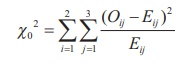This chi-square test statistic is calculated as follows:= 22.152 with degrees of freedom (3ŌĆō1)(2ŌĆō1) = 2

Step 5 : Critical value

From  the chi-square  table  the  critical  value  at  5%  level  of  significance  is Žć2 (2-1)(3-1), 0.05 = Žć 22 , 0.05 = 5.991.

Step 6 : Decision

As the calculated value Žć02 = 22.152 is greater than the critical value Žć2 2005 = 5.991 the null hypothesis H0 is rejected. Hence, the performance and age of students are not independent.

The following example will illustrate the procedure

### Example 2.10

A survey was conducted with 500 female students of which 60% were intelligent, 40% had uneducated fathers, while 30 % of the not intelligent female students had educated fathers. Test the hypothesis that the education of fathers and intelligence of female students are independent.

### Solution:

Step 1 : Null hypothesis H0: The attributes are independent i.e. No association between education fathers and intelligence of female students

Alternative hypothesis H1: The attributes are not independent i.e there is association between education of fathers and intelligence of female students

Step 2 : Data

The observed frequencies (O) has been computed from the given information as under.Step 3 : Level of significance

╬▒ = 5%

Step 4 : Calculation

Calculate the value of the test statistic aswhere, a= 620, b = 380, c = 550, d = 450 and N = 2000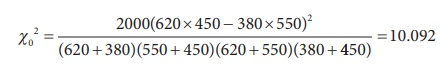Step 5 : Critical value

From chi-square table the critical value at 5% level of significance is  Žć 21, 0.05 = 3.841

Step 6 : Decision

The calculated value  Žć 20  = 10.092 is greater than the critical value   Žć 21, 0.05 = 3.841, the null hypothesis H0 is rejected. Hence, education of fathers and intelligence of female students are not independent.

## 6. Tests for Goodness of Fit

Another important application of chi-square distribution is testing goodness of a pattern or distribution fitted to given data. This application was regarded as one of the most important inventions in mathematical sciences during 20th century. Goodness of fit indicates the closeness of observed frequency with that of the expected frequency. If the curves of these two distributions do not coincide or appear to diverge much, it is noted that the fit is poor. If two curves do not diverge much, the fit is fair.

## Computational steps for testing the significance of goodness of fit:

Step 1 : Framing of hypothesis

Null hypothesis H0 : The goodness of fit is appropriate for the given data set

Alternative hypothesis H1 : The goodness of fit is not appropriate for the given data set

Step 2 : Data

Calculate the expected frequencies (Ei) using appropriate theoretical distribution such as Binomial or Poisson.

Step 3 : Select the desired level of significance ╬▒

Step 4 : Test statistic

The test statistic is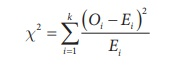where k = number of classes

Oi and Ei are respectively the observed and expected frequency of ith class such thatIf any of Ei is found less than 5, the corresponding class frequency may be pooled with preceding or succeeding classes such that Ei's of all classes are greater than or equal to 5. It may be noted that the value of k may be determined after pooling the classes.

The approximate sampling distribution of the test statistic under H0 is the chi-square distribution with k-1-s d.f , s being the number of parametres to be estimated.

Step 5 : Calculation

Calculate the value of chi-square asThe above steps in calculating the chi-square can be summarized in the form of the table as follows:

Step 6 : Critical value

The critical value is obtained from the table of Žć2 for a given level of significance ╬▒.

Step 7 : Decision

Decide on rejecting or not rejecting the null hypothesis by comparing the calculated value of the test statistic with the table value, at the desired level of significance.

Example 2.11

Five coins are tossed 640 times and the following results were obtained.Fit binomial distribution to the above data.

Solution:

Step 1 : Null hypothesis H0: Fitting of binomial distribution is appropriate for the given data.

Alternative hypothesis H1: Fitting of binomial distribution is not appropriate to the given data.

Step 2 : Data

Compute the expected frequencies:

n = number of coins tossed at a time = 5

Let X denote the number of heads (success) in n tosses

N = number of times experiment is repeated = 640

To find mean of the distributionThe probability mass function of binomial distribution is :

p(x) = nCx px qnŌĆōx, x = 0,1,..., n                            (2.1)

Mean of the binomial distribution is= np.For x = 0, the equation (2.1) becomes

P(X = 0) = P(0) = 5c0 (0.5)5 = 0.03125

The expected frequency at x = N P(x)

The expected frequency at x =0 : N ├Ś P(0)

= 640 ├Ś 0.03125 = 20

We use recurrence formula to find the other expected frequencies.

The expected frequency at x+1 is├Ś Expected frequency at x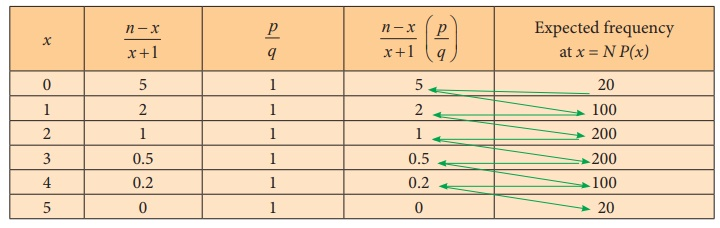Table of expected frequencies: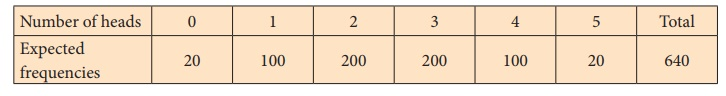Step 3 : Level of significance

╬▒= 5%

Step 4 : Test statisticStep 5 : Calculation

The test statistic is computed as under:Step 6 : Critical value

Degrees of freedom = k ŌĆō 1 ŌĆō s = 6 ŌĆō 1 ŌĆō 1 = 4

Critical value for d.f 4 at 5% level of significance is 9.488 i.e., c42, 0.05 = 9.488

Step 7 : Decision

As the calculated Žć 0 2 (=0.575) is less than the critical value  Žć24, 0.05   = 9.488, we do not reject the null hypothesis. Hence, the fitting of binomial distribution is appropriate.

Example 2.12

A packet consists of 100 ball pens. The distribution of the number of defective ball pens in each packet is given below:Examine whether Poisson distribution is appropriate for the above data at 5% level of significance.

Solution:

Step 1 : Null hypothesis H0: Fitting of Poisson distribution is appropriate for the given data.

Alternative hypothesis H1: Fitting of Poisson distribution is not appropriate for the given data.

Step 2 : Data

The expected frequencies are computed as under:

To find the mean of the distribution.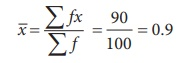Probability mass function of Poisson distribution is:In the case of Poisson distribution mean (m) == 0.9.

At x = 0, equation (2.2) becomesThe expected frequency at x is N P(x)

Therefore, The expected frequency at 0 is

N ├Ś P (0)

= 100 ├Ś 0.4066

= 40.66

We use recurrence formula to find the other expected frequencies.

The expected frequency at x+1 is

[ m  / x+1 ] ├Ś Expected frequency at xTable of expected frequency distribution (on rounding to the nearest integer)Step 3 : Level of significance

╬▒ = 5%

Step 4 : Test statistic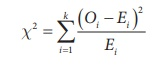Step 5 : Calculation

The test statistic is computed as under:Note: In the above table, we find the cell frequencies 0,1 in the expected frequency column (E) is less than 5, Hence, we combine (pool) with either succeeding or preceding one such that the total is made greater than 5. Here we have pooled with preceding frequency 5 such that the total frequency is made greater than 5. Correspondingly, cell frequencies in observed frequencies are pooled.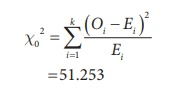Step 6 : Critical value

Degrees of freedom = (k ŌĆō 1 ŌĆō s) = 4 ŌĆō 1 ŌĆō 1 = 2

Critical value for 2 d.f at 5% level of significance is 5.991 i.e., Žć22, 0.05 = 5.991

Step 7 : Decision

The calculated Žć02 (=51.253) is greater than the critical value (5.991) at 5% level of significance. Hence, we reject H0. i.e., fitting of Poisson distribution is not appropriate for the given data.

Example 2.13

A sample 800 students appeared for a competitive examination. It was found that 320 students have failed, 270 have secured a third grade, 190 have secured a second grade and the remaining students qualified in first grade. The general opinion that the above grades are in the ratio 4:3:2:1 respectively. Test the hypothesis the general opinion about the grades is appropriate at 5% level of significance.

Step 1 : Null hypothesis H0: The result in four grades follows the ratio 4:3:2:1

Alternative hypothesis H1: The result in four grades does not follows the ratio 4:3:2:1

Step 2 : Data

Compute expected frequencies:

Under the assumption on H0, the expected frequencies of the four grades are:

4/10 ├Ś 800 = 320 ; 3/10 ├Ś 800 = 240; 2/10 ├Ś 800 = 160; 1/10 ├Ś 800 =80

Step 3 : Test statistic

The test statistic is computed using the following table.The test statistic is calculated asStep 4 : Critical value

The critical value of Žć2 for 3 d.f. at 5% level of significance is 7.81 i.e., Žć 23, 0.05 = 7.81

Step 5 : Decision

As the calculated value of Žć 02 (=54.375) is greater than the critical value Žć2 3, 0.05 = 7.81, reject H0. Hence, the results of the four grades do not follow the ratio 4:3:2:1.

Example 2.14

The following table shows the distribution of digits in numbers chosen at random from a telephone directory.Test whether the occurence of the digits in the directory are equal at 5% level of significance.

Step 1 : Null hypothesis H0: The occurrence of the digits are equal in the directory.

Alternative hypothesis H1: The occurrence of the digits are not equal in the directory.

Step 2 : Data

The expected frequency for each digit = 10000/10 = 1000

Step 3 : Level of significance

╬▒ = 5%

Step 4 : Test statistic

The test statistic is computed using the following table.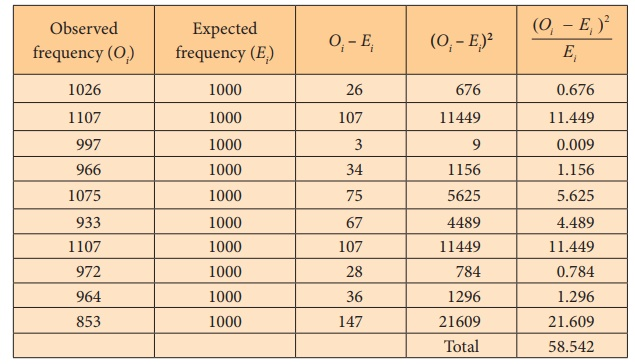The test statistic is calculated asStep 4  :  Critical value

Critical value for 9 df at 5% level of significance is 16.919 i.e., Žć29, 0.05 = 16.919

Step 5  :  Decision

Since the calculated Žć02 (58.542) is greater than the critical value Žć29, 0.05 = 16.919, reject H0. Hence, the digits are not uniformly distributed in the directory.

Tags : Properties, Procedure Steps, Example Solved Problems | Statistics , 12th Statistics : Chapter 2 : Tests Based on Sampling Distributions I
Study Material, Lecturing Notes, Assignment, Reference, Wiki description explanation, brief detail
12th Statistics : Chapter 2 : Tests Based on Sampling Distributions I : Chi-Square Distribution and Its Applications | Properties, Procedure Steps, Example Solved Problems | Statistics